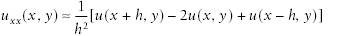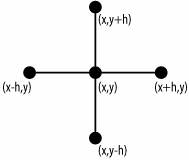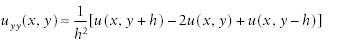# Leveraging Excel to Directly Solve Finite Difference Equations

Problem

You've formulated the finite difference equations for an elliptic boundary value problem and now you need to solve the resulting system of equations.

Solution

Use Excel's matrix functions to solve the system of finite difference equations.

Discussion

The finite difference method is a well-known and well-established numerical method suitable for solving partial differential equations. Virtually every book I've ever read dealing with partial differential equations covers the finite difference method to some degree. DuChateau and Zachmann give a pretty good introduction, with examples, on the application of the finite difference method to partial differential equations in their book Applied Partial Differential Equations.[*]

[*] Paul DuChateau and David Zachmann, Applied Partial Differential Equations (Mineola, N.Y.: Dover Publications, 2002; originally published 1989 by Harper & Row).

The basic idea of the finite difference method is to divide the problem domain into a finite number of cells, forming a grid. The intersections of grid lines then represent computational nodes where the value of the function sought will be approximated. The governing differential equation is then written in terms of finite differences (see Recipe 10.6). These finite differences could be forward differences, backward differences, or central differences, and they are used to approximate the derivatives appearing in the governing partial differential equations at each node in the computational grid.

Consider the Laplace equation :If we discretize the problem domain as describe a moment ago, then at each node illustrated in Figure 12-1, we can approximate the second partial derivative of u with respect to x using the following finite difference approximation:Figure 12-1. Finite difference nodesSimilarly, the finite difference approximation for uyy is:Here, I'm assuming the node spacing is uniform and the same in both the x and y directions. Substitution of these finite difference approximations into the Laplace equation yields the following finite difference equation:This equation must be satisfied at every node. Nodes adjacent to boundary nodes with Dirichlet boundary conditions will include the specified value at the boundary node. Typically, a ghost particle technique is used in the case of Neumann boundary conditions. In any case, writing this finite difference approximation at each node in the computation grid results in a system of equations that must be solved to find the u-values at each node. It's this resulting system of equations that can be solved in Excel.

For example, a rectangular domain divided into nine equal-sized cells would contain four interior nodes where values of u are sought. Applying the above finite difference equation at each node would result in a system of four equations and four unknowns. In this case, with such a small number of equations, you can use matrix formulas discussed in Recipe 9.4 to solve for the unknown u-values.

Finer computational grids, or meshes, result in a larger number of nodes and thus a larger system of equations. In these cases, it's usually more efficient and easier to use iterative methods to solve the system. Recipe 12.2 explains such an approach.

The body of literature covering finite difference methods is vast. Consult your favorite numerical methods book for more information. I found the Applied Partial Differential Equations book cited earlier in this recipe very readable and comprehensive in its coverage of the finite difference method to elliptic, parabolic, and hyperbolic problems.Excel Scientific and Engineering Cookbook (Cookbooks (OReilly))
ISBN: 0596008791
EAN: 2147483647
Year: N/A
Pages: 206
Authors: David M Bourg